# 11. Input devices¶

This week I worked on in reading data from RPLIDAR A1M8-R6 - 360 Degree Laser Scanner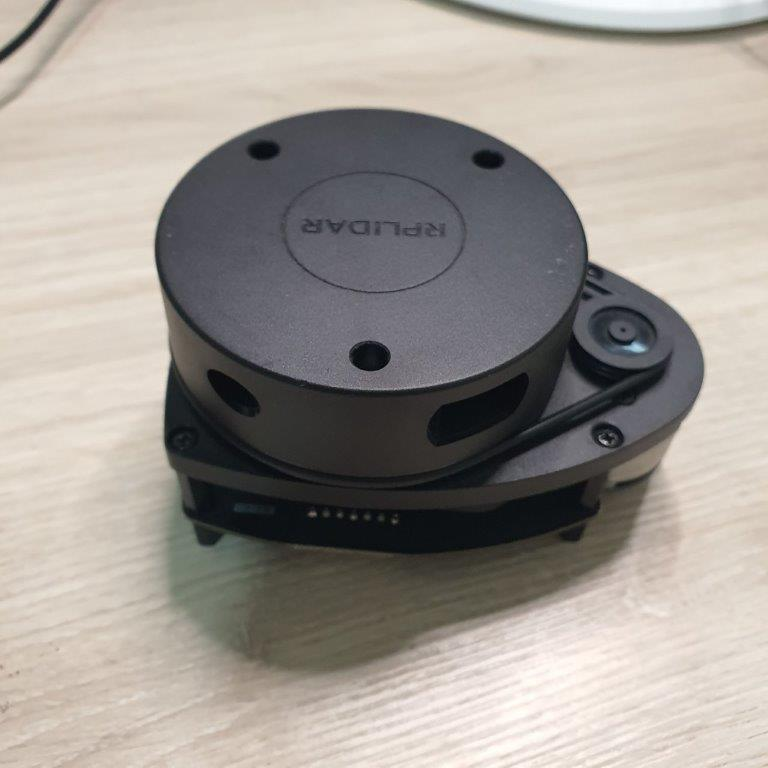In the following video the LED will LED blink faster when the obstacle get closer to the sensor.

### Wiring¶

My Board Pin RPLidar
FTDI GND GND
FTDI RX RX
FTDI TX TX
VCC V5.0
GND GND
FTDI CTS MOTOCTL
VCC VMOTO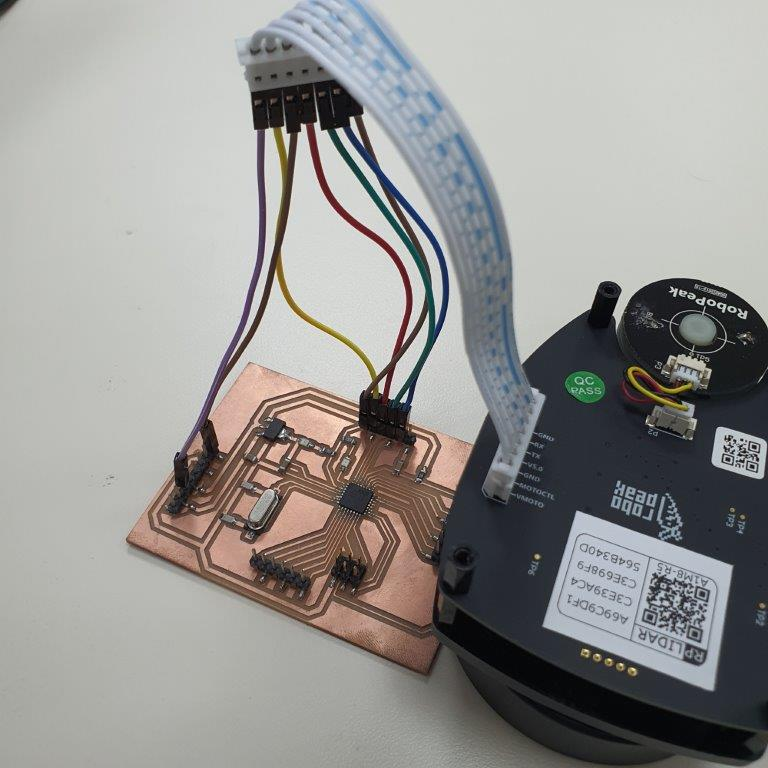• For programing I wire Arduino UNO board with ISP header of my board
Arduino UNO Pin # New Board ISP Port
12 1-MISO
5V 2-VCC
13 3-SCK
11 4-MOSI
10 5-RST
GND 6-GND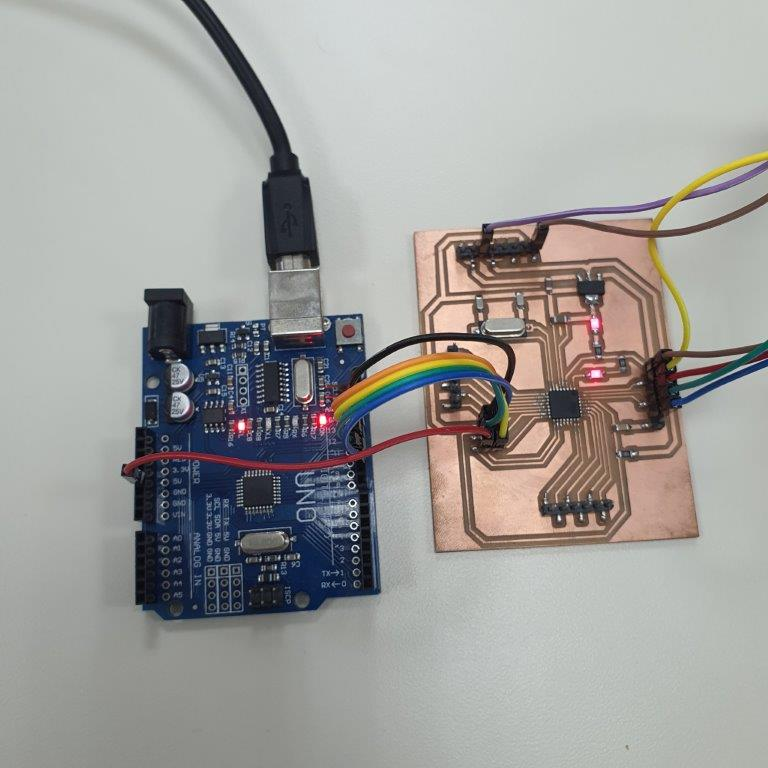• Here is the full wiring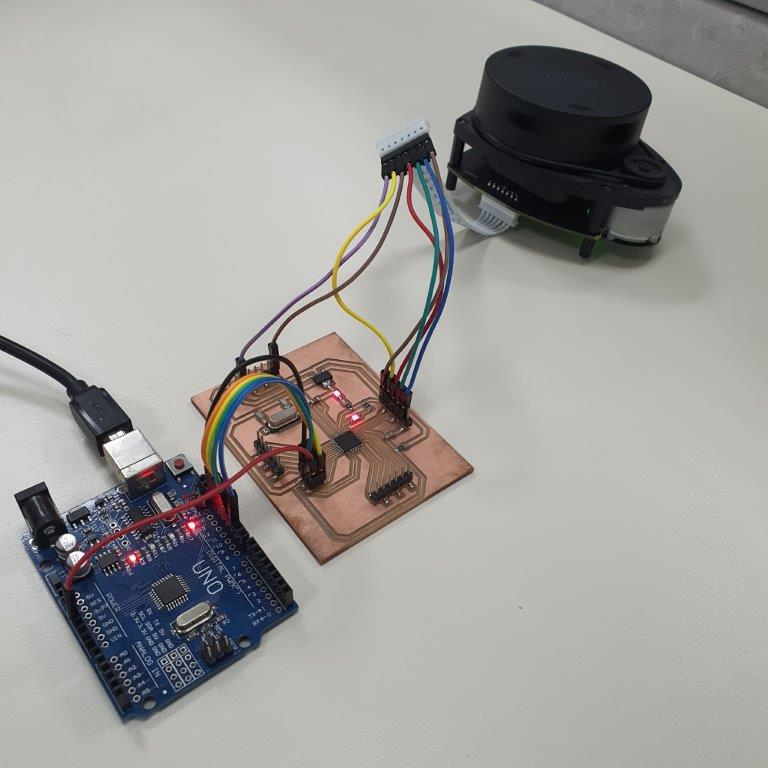### RPLIDAR library¶

• First you’ll need to install the RPLIDAR library from GitHub by download the ZIP file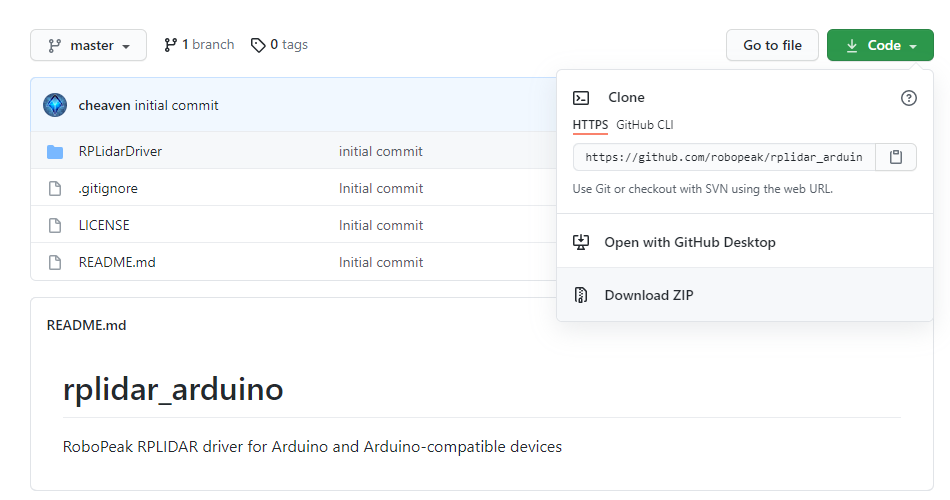• Export RPLidarDriver folder to Arduino libraries folder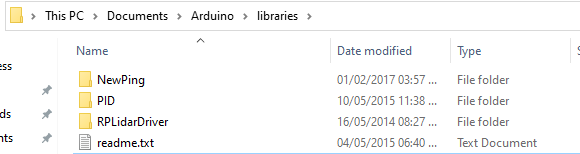### Arduino Code¶

• Open Arduino IDE and go to File >> Examples >> RPLidarDriver >> simple_connect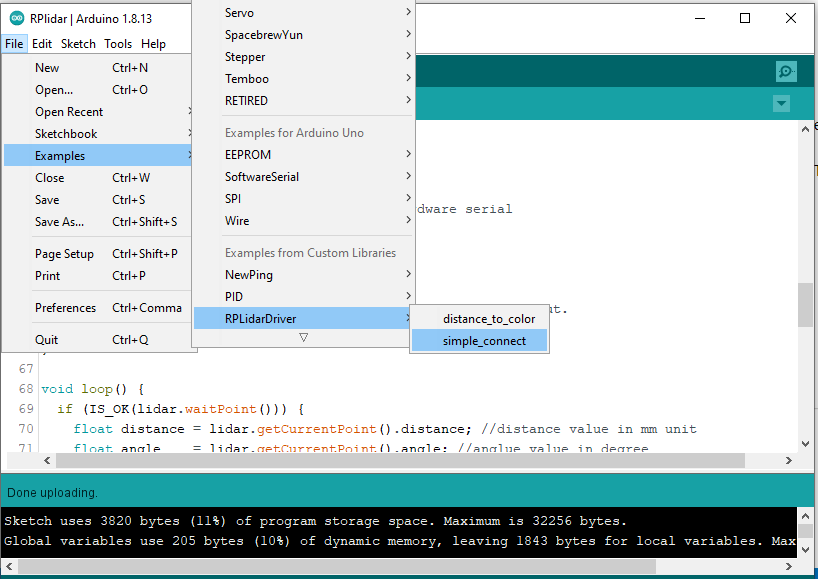• I modify the code by adding the following code

``````      //perform data processing here...

if (angle >= 0.0 && angle <= 1.0) {
if ( distance <= 150 && distance > 100)
interval = 500;
else if ( distance <= 100 && distance > 50)
interval = 100;
else if ( distance <= 50 && distance >= 0)
interval = 0;
else
interval = 2000;

timeNow = millis();
if ( (timeNow - timeOld) >= interval) {
timeOld = timeNow;
if (LEDStatus == LOW)
LEDStatus = HIGH;
else
LEDStatus = LOW;
}
digitalWrite(pinLED, LEDStatus);
}
``````
• Here is the full code
``````// This sketch code is based on the RPLIDAR driver library provided by RoboPeak
#include <RPLidar.h>

// You need to create an driver instance
RPLidar lidar;

#define RPLIDAR_MOTOR 2 // The PWM pin for control the speed of RPLIDAR's motor.
// This pin should connected with the RPLIDAR's MOTOCTRL signal

float distanceOld = 0;
unsigned long timeNow = millis();
unsigned long timeOld = millis();
unsigned long interval = 0;
int pinLED = 3;
byte LEDStatus = LOW;

void setup() {
// bind the RPLIDAR driver to the arduino hardware serial
lidar.begin(Serial);

// set pin modes
pinMode(RPLIDAR_MOTOR, OUTPUT);
pinMode(pinLED, OUTPUT); // initialize digital pin as an output.
digitalWrite(pinLED, HIGH);
}

void loop() {
if (IS_OK(lidar.waitPoint())) {
float distance = lidar.getCurrentPoint().distance; //distance value in mm unit
float angle    = lidar.getCurrentPoint().angle; //anglue value in degree
bool  startBit = lidar.getCurrentPoint().startBit; //whether this point is belong to a new scan
byte  quality  = lidar.getCurrentPoint().quality; //quality of the current measurement

//perform data processing here...

if (angle >= 0.0 && angle <= 1.0) {
if ( distance <= 150 && distance > 100)
interval = 500;
else if ( distance <= 100 && distance > 50)
interval = 100;
else if ( distance <= 50 && distance >= 0)
interval = 0;
else
interval = 2000;

timeNow = millis();
if ( (timeNow - timeOld) >= interval) {
timeOld = timeNow;
if (LEDStatus == LOW)
LEDStatus = HIGH;
else
LEDStatus = LOW;
}
digitalWrite(pinLED, LEDStatus);
}
} else {
analogWrite(RPLIDAR_MOTOR, 0); //stop the rplidar motor

// try to detect RPLIDAR...
rplidar_response_device_info_t info;
if (IS_OK(lidar.getDeviceInfo(info, 100))) {
// detected...
lidar.startScan();

// start motor rotating at max allowed speed
analogWrite(RPLIDAR_MOTOR, 255);
delay(1000);
}
}
}
``````

### Testing¶

In the following video the LED will LED blink faster when the obstacle get closer to the sensor.

## ROS¶

Last update: June 16, 2021